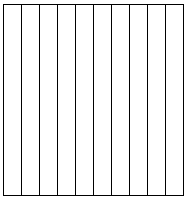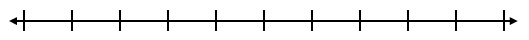# Decimal Fractions

## Objective

Represent decimals to hundredths less than one, understanding the equivalence of some number of tenths and 10 times as many hundredths. Convert between fraction, decimal, and unit form.

## Common Core Standards

### Core Standards

?

• 4.NF.C.5 — Express a fraction with denominator 10 as an equivalent fraction with denominator 100, and use this technique to add two fractions with respective denominators 10 and 100. Students who can generate equivalent fractions can develop strategies for adding fractions with unlike denominators in general. But addition and subtraction with unlike denominators in general is not a requirement at this grade. For example, express 3/10 as 30/100, and add 3/10 + 4/100 = 34/100.

• 4.NF.C.6 — Use decimal notation for fractions with denominators 10 or 100. For example, rewrite 0.62 as 62/100; describe a length as 0.62 meters; locate 0.62 on a number line diagram.

?

• 3.NF.A.2

• 4.NF.A.1

## Criteria for Success

?

1. Understand hundredths as a further extension of the place value system to represent values that are 1 tenth of 1 tenths (or 1 hundredth of 1) (MP.7)
2. Explain why some number of tenths is equivalent to ten times as many hundredths, using a visual model or multiplication/division to demonstrate this understanding (MP.3).
3. Represent decimals to hundredths less than one written in decimal, fraction, or unit form using an area model (MP.5).
4. Represent decimals to hundredths less than one written in decimal, fraction, or unit form using a number line (MP.5).
5. Convert between fraction form, decimal form, and unit form for some number of hundredths less than one.

## Tips for Teachers

?

• The following material is needed for today's lesson: base ten blocks
• “There are several ways to read decimals aloud. For example, 0.15 can be read as “1 tenth and 5 hundredths” or “15 hundredths,” just as 1,500 is sometimes read “15 hundred” or “1 thousand, 5 hundred” (NF Progression, p. 15). In your own language use, opt for the former way of saying a decimal, since this is more common and because reading each place value separately will become cumbersome (e.g., “4.583” would be read as “four and 5 tenths 8 hundredths 3 thousandths” rather than ‘four and 583 thousandths”). While “mathematicians and scientists often read 0.15 aloud as ‘zero point one five’ or ‘point one five,’” refrain from using this language until you are sure students have a strong sense of place value with decimals (NF Progression, p. 15).

#### Remote Learning Guidance

If you need to adapt or shorten this lesson for remote learning, we suggest prioritizing Anchor Task 2 (benefits from worked example) and Anchor Task 3 (benefits from discussion). Find more guidance on adapting our math curriculum for remote learning here.

#### Fishtank Plus

• Problem Set
• Student Handout Editor
• Vocabulary Package

?

### Problem 1

1. Complete the following sentences.
1. 1 thousand is the same as _______ hundreds.
2. 1 hundred is the same as _______ tens.
3. 1 ten is the same as _______ ones.
4. 1 one is the same as _______ tenths.
2. Use what you notice in (a) to write a similar sentence that starts “1 tenth is the same as…”
3. Use what you just learned in (a) and (b) above to fill in the missing place value.### Problem 2

a. Below is an area model that represents 1 one. It is partitioned into tenths. How would you partition it further to represent hundredths?b. Use the area model above to represent 7 hundredths. Then represent 7 hundredths in fraction and decimal form.

### Problem 3

1. Explain why ${{1\over10}={10\over100}}$. Draw a picture to illustrate your explanation.
2. Explain why ${0.20 = 0.2}$. Draw a picture to illustrate your explanation.

#### References

Illustrative Mathematics Fraction Equivalence

Fraction Equivalence, accessed on May 29, 2018, 11:07 a.m., is licensed by Illustrative Mathematics under either the CC BY 4.0 or CC BY-NC-SA 4.0. For further information, contact Illustrative Mathematics.

Modified by The Match Foundation, Inc.

### Problem 4

Jani and Kai are representing a decimal number. Here are the number lines they drew:

 JaniKai1. What value do you think Jani and Kai are trying to model? How do you know?
2. How do you think Jani and Kai would have used area models to model the same value?
3. Use an area model and a number line to model the following values. Then write them in fraction form and decimal form.
1. 48 hundredths
2. 5 tenths 1 hundredth
3. 70 hundredths

## Problem Set & Homework

#### Discussion of Problem Set

• Vivian thinks that the decimal represented on the number line in #2c is 0.3. Zach says it is 0.30. Who is correct? How do you know?
• What did you notice about the number line in #2e?
• How did the models in #4 show that 0.3 = 0.30?
• What decimal was modeled in #5d? How do you know? How was this area model different from the previous ones?
• What are two ways you could have modeled ${{6\over10}}$ in #6a?
• Compare the answers to #7d and #7f. Did you write the same equivalent numbers? Why are there several possibilities for answers in these two problems? Where have we seen that before?

?

### Problem 1

a. Model 53 hundredths on the area model below.b. What is 53 hundredths written as a decimal?

### Problem 2

a.  Plot the point ${{{76\over100}}}$ on the number line below. Make sure to label the endpoints.b.  What is ${{{76\over100}}}$ written as a decimal?

### Problem 3

Damien says 0.78 is equivalent to 78 hundredths. Kelly says 0.78 is equivalent to 7 tenths and 8 hundredths. Who is right? Explain how you know.

### Mastery Response

?